## Solutions to the Monge-Ampere Equation with Polyhedral and Y-shaped Singularities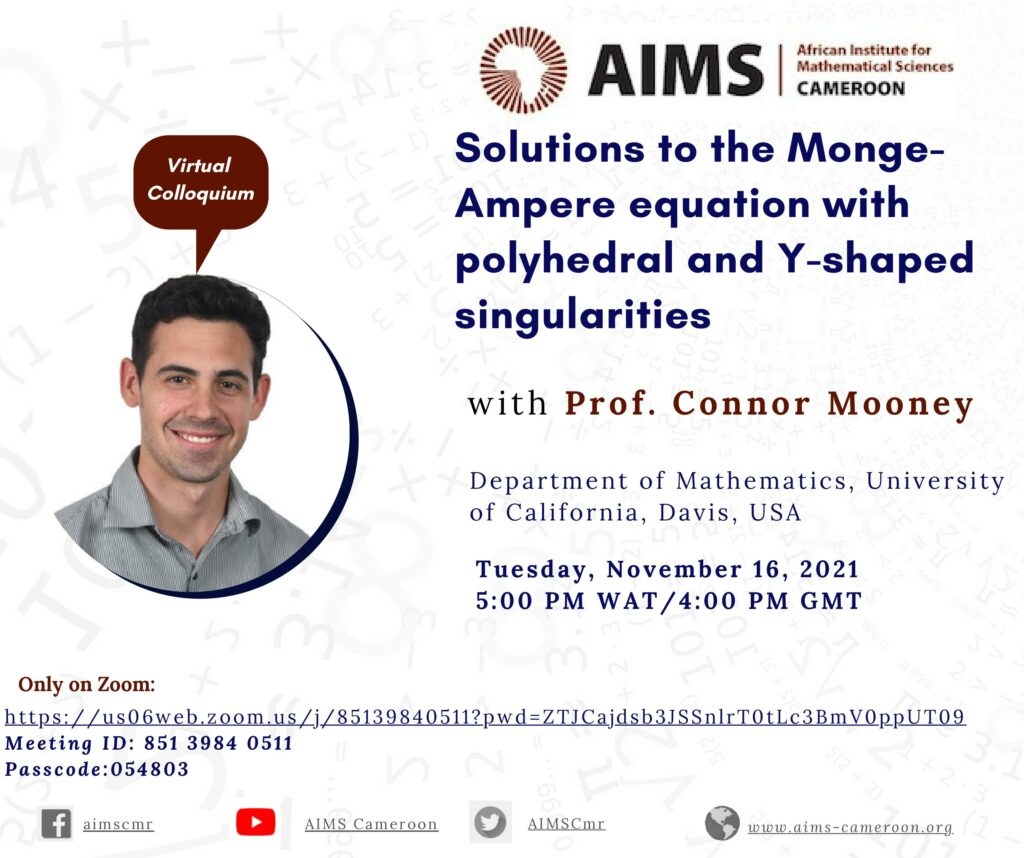Surfaces which The Monge-Ampere equation $\mathrm{det}(D^2u) = 1$ arises in prescribed curvature problems and in optimal transport. An interesting feature of the equation is that it admits singular solutions. We will discuss new examples of convex functions on $\mathbb{R}^n$ that solve the Monge-Ampere equation away from finitely many points but contain polyhedral and $Y$-shaped singular structures. Along the way, we will discuss

## The Stickiness Property of Nonlocal Minimal Surfaces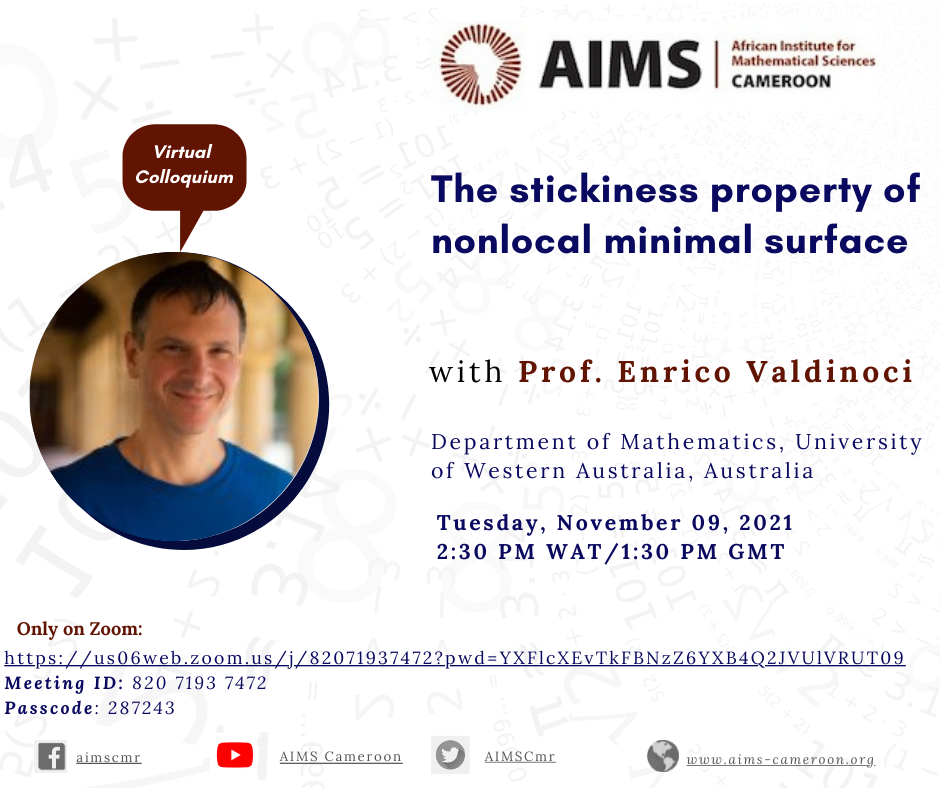Surfaces that minimize a nonlocal perimeter functional exhibit quite different behaviors than the ones minimizing the classical perimeter. Among these peculiar features, an interesting property, which is also in contrast with the pattern produced by the solutions of linear equations, is given by the capacity, and the strong tendency, of adhering at the boundary. We

## Generic Regularity in the Fractional Obstacle Problem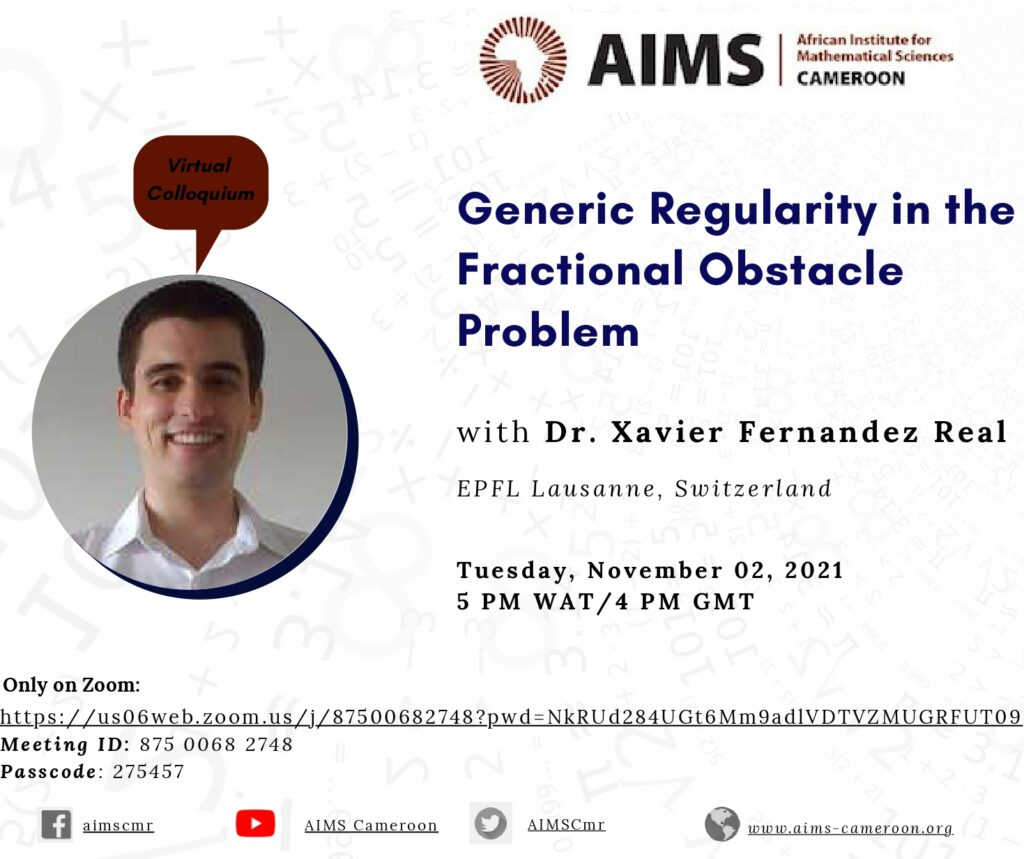The fractional obstacle problem in $\mathbb R^n$ with obstacle $\varphi\in C^\infty(\mathbb{R}^n)$ can be written as $\min\{(-\Delta)^s u , u-\varphi\} = 0,\quad\textrm{in }\quad\mathbb{R}^n.$ The set $\{u = \varphi\} \subset \mathbb{R}^n$ is called the contact set, and its boundary is the free boundary, an unknown of the problem. The free boundary for the fractional obstacle problem can be divided between two subsets: regular points

## Well-posedness of Non-Linear Partial Differential Equations in Weighted Sobolev Spaces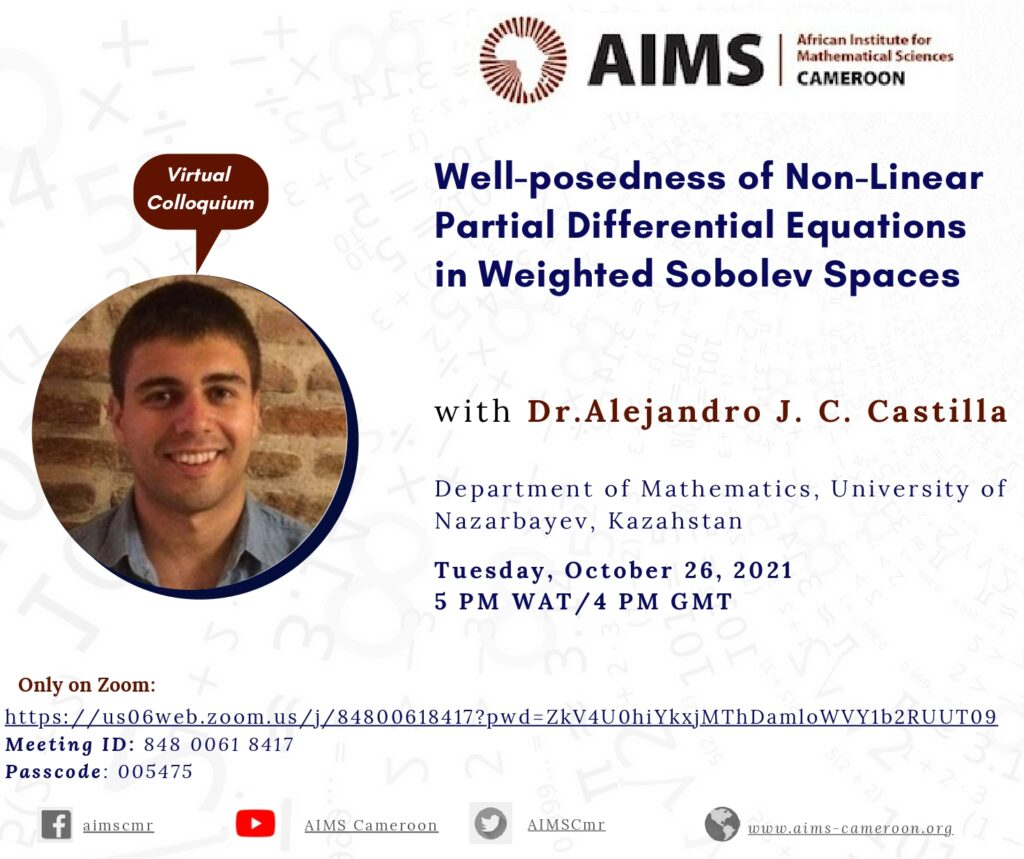The well-posedness (existence, uniqueness, and stability) of the solutions for several nonlinear dispersive PDEs with initial data in Sobolev spaces has been intensively analyzed. However, Sobolev functions do not necessarily decay fast at infinity, which is in many applications an unrealistic assumption. In this talk, we discuss examples of existence and uniqueness results in weighted

## Maiden Edition of the Aims-Cameroon Mathematics and its Applications Meeting (ACMAM) Holds in January 2022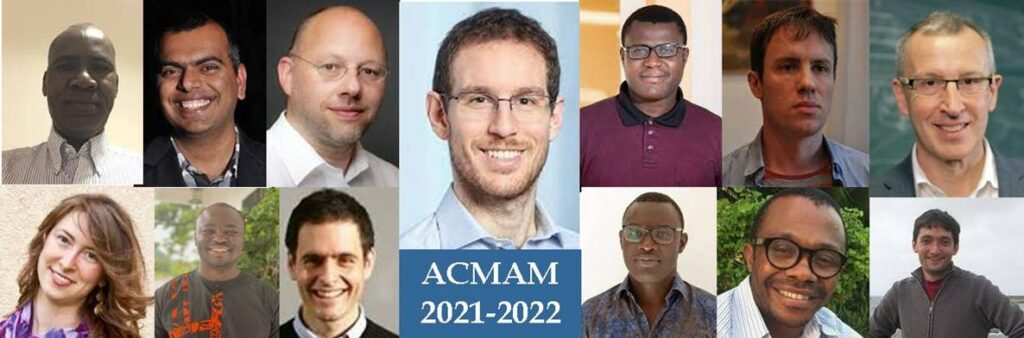AIMS Cameroon Research Center will organize the maiden edition of its annual scientific event named AIMS-Cameroon Mathematics and its Applications Meeting (ACMAM) from the 12th to the 14th of January, 2022. Lectures take place at the AIMS Cameroon Research Center in Limbe South West Region. The AIMS-Cameroon Mathematics and its Applications Meeting (ACMAM) is an

## Regularity for C^{1,\alpha} interface transmission problems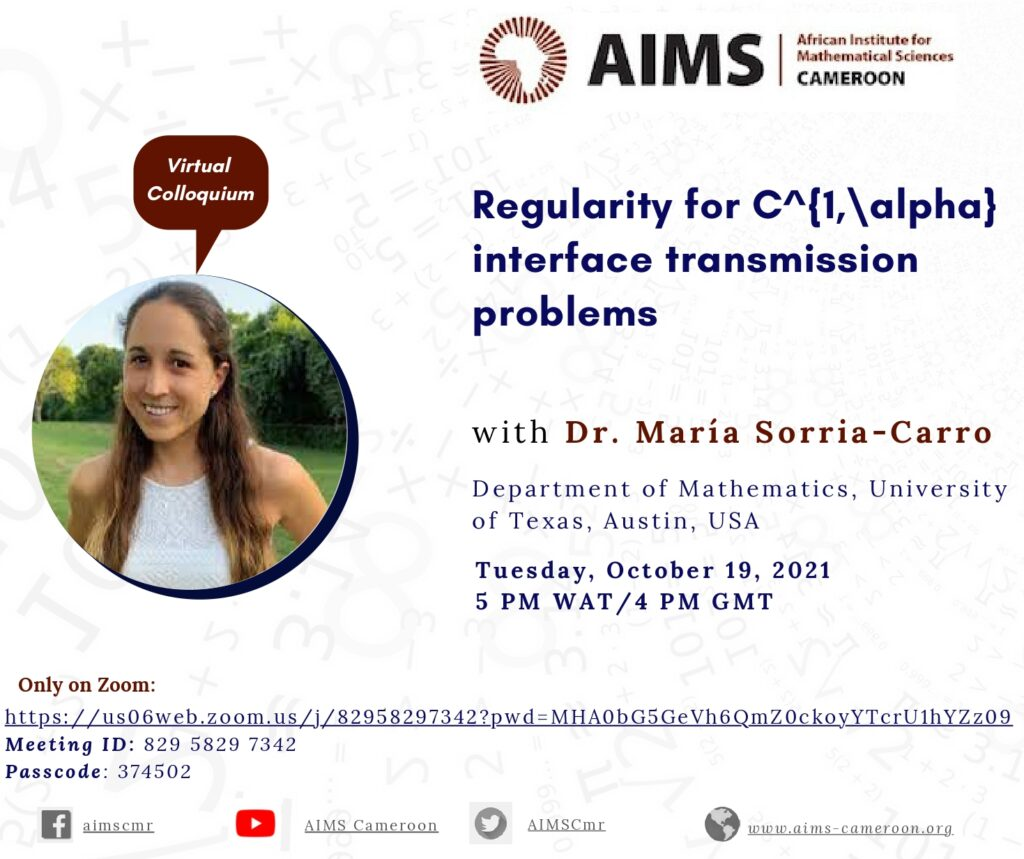Transmission problems originally arose in elasticity theory, and they are nowadays of great interest due to their many applications in different areas in science. Typically, in these problems, there is a fixed interface where solutions may change abruptly, and the primary focus is to study their behavior across this surface. In this talk, we will

## Regularity Estimate for Nonlocal Space-Time Master Equations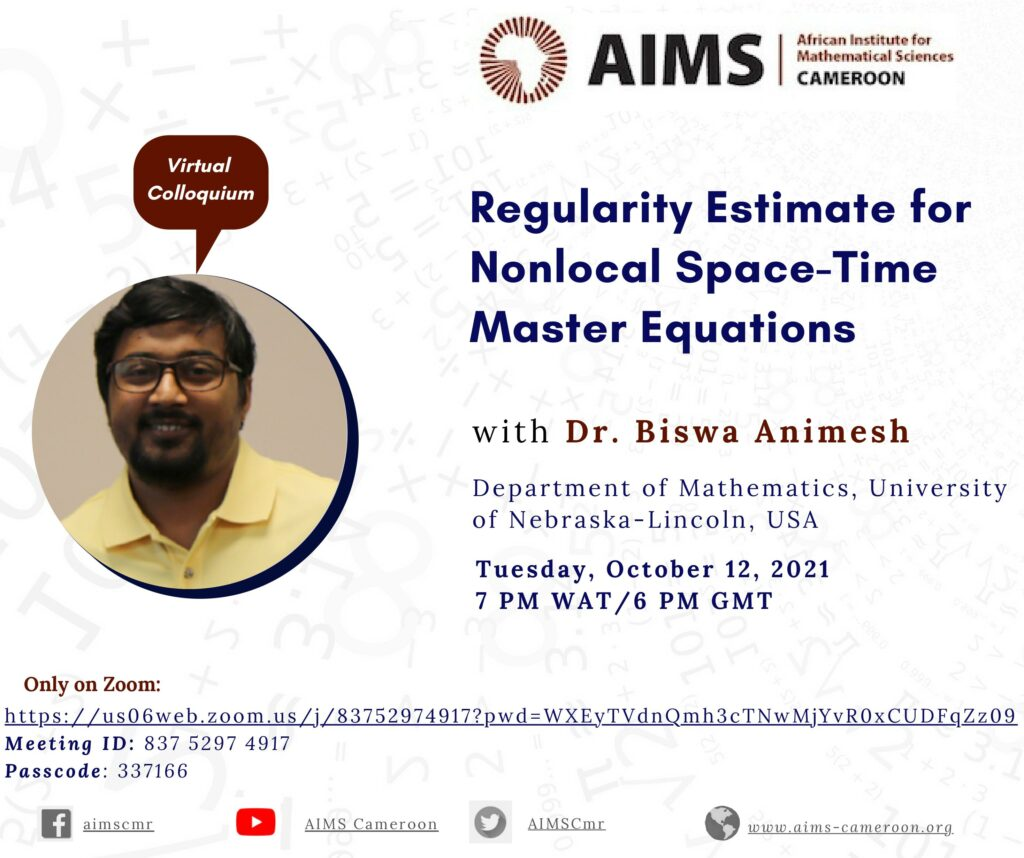We analyze regularity estimates for solutions to nonlocal space-time equations driven by fractional powers of parabolic operators in divergence form. These equations are fundamental in semipermeable membrane problems, biological invasion models and they also appear as generalized Master equations. We develop a parabolic method of semigroups that allows us to prove a local extension problem

## Instability of Finite Time Blow-up For Incompressible Euler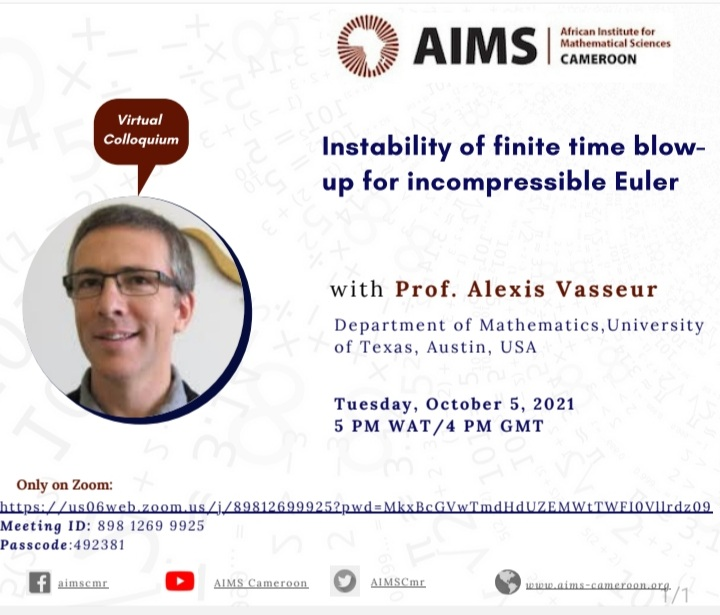In this talk, we will discuss the interaction between the stability, and the propagation of regularity, for solutions to the incompressible 3D Euler equation. It is still unknown whether a solution with smooth initial data can develop a singularity in finite time. We will describe how, in such a scenario, the solution becomes unstable as

## Boundedness Properties for Hermite Pseudo-Multipliers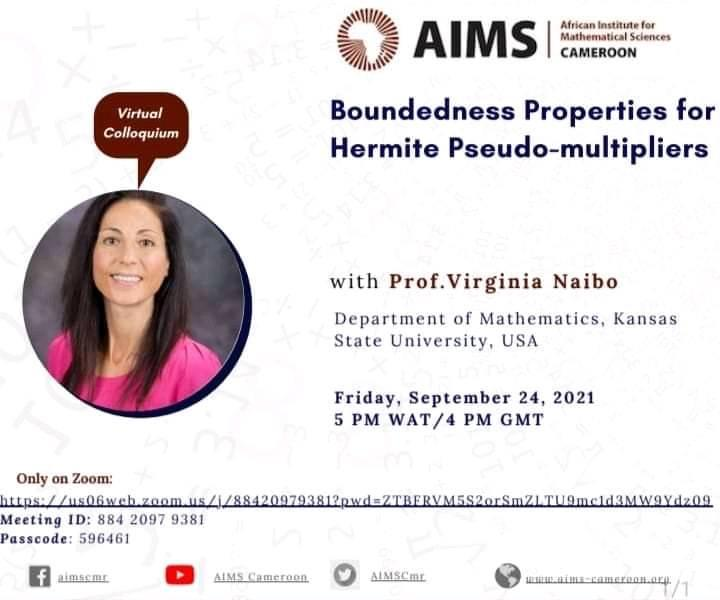Fourier multipliers and pseudo-differential operators are defined by means of the Fourier transform and play an important role in the study of partial differential equations. In the same spirit, Hermite pseudo-multipliers are associated to Hermite expansions and they represent the counterparts to pseudo-differential operators in the Hermite setting. After some preliminaries, we will present results

## Sharp Quantitative Faber-Krahn inequalities and the Alt-Caffarelli-Fredman Monotonicity Formula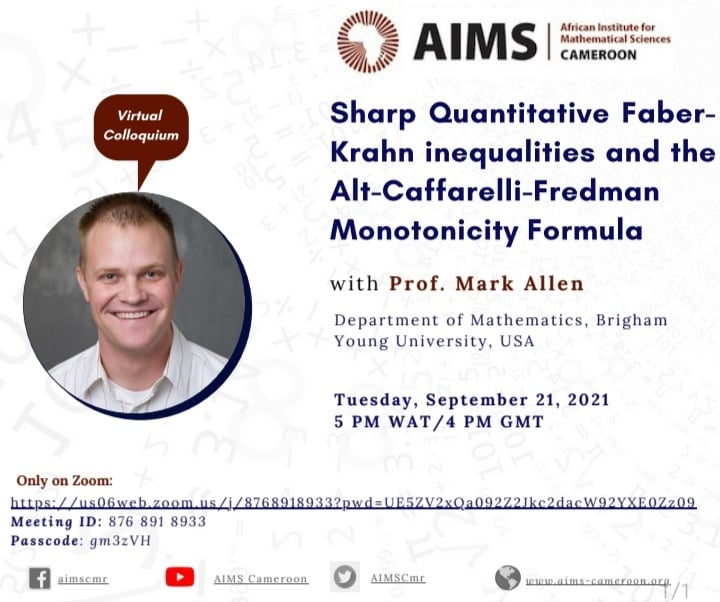The Colloquium will be focused on this research work by Mark Allen of Brigham Young University in the USA. He establishes the new sharp quantitative estimates for Faber-Krahn inequalities on Euclidean space, the round sphere and hyperbolic space and then applies these inequalities in order to establish a quantitative form of the Alt-Caffarelli-Friedman monotonicity formula.